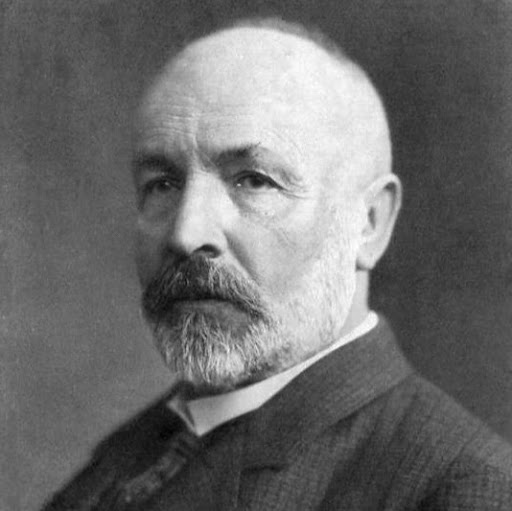# Unknown x

$x^o$N, $y^o$ELearning to think :)Top Questions

## $\sum\limits_{n=1}^\infty \log(1+a_n)$ converges absolutely $\iff\sum\limits_{n=1}^\infty a_n$ converges absolutely.

asked Oct 10 '17 at 16:52

## $S$ be a non empty subset of $\mathbb{R}$ having supremum and $T=\{x\in\mathbb{R}: x-a \in S\}$, then $\forall a \in \mathbb{R}$, $\sup T=a+\sup S$.

asked Aug 6 '17 at 2:00

## Let $x_1=a>0$ and $x_{n+1}=x_n+\frac{1}{x_n} \forall n\in \mathbb N$. Check whether the following sequnce converges or diverges.

asked Apr 29 '18 at 5:14